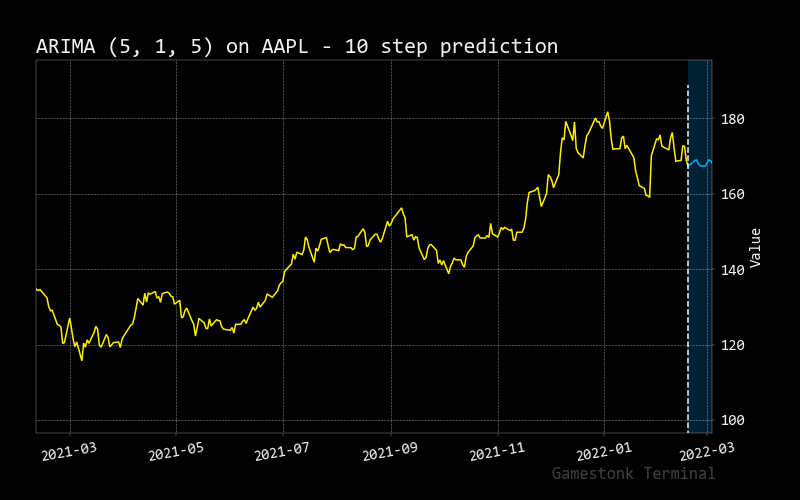# Arima

``````usage: arima [-d N_DAYS] [-i {aic,aicc,bic,hqic,oob}] [-s] [-o S_ORDER] [-r] [-e S_END_DATE] [-h]
[--export {png,jpg,pdf,svg}]
``````

In statistics and econometrics, and in particular in time series analysis, an autoregressive integrated moving average (ARIMA) model is a generalization of an autoregressive moving average (ARMA) model. Both of these models are fitted to time series data either to better understand the data or to predict future points in the series (forecasting). ARIMA(p,d,q) where parameters p, d, and q are non-negative integers, p is the order (number of time lags) of the autoregressive model, d is the degree of differencing (the number of times the data have had past values subtracted), and q is the order of the moving-average model.

``````optional arguments:
-d N_DAYS, --days N_DAYS
prediction days. (default: 5)
-i {aic,aicc,bic,hqic,oob}, --ic {aic,aicc,bic,hqic,oob}
information criteria. (default: aic)
-s, --seasonal        Use weekly seasonal data. (default: False)
-o S_ORDER, --order S_ORDER
arima model order (p,d,q) in format: p,d,q. (default: )
-r, --results         results about ARIMA summary flag. (default: False)
-e S_END_DATE, --end S_END_DATE
The end date (format YYYY-MM-DD) to select - Backtesting (default: None)
-h, --help            show this help message (default: False)
--export {png,jpg,pdf,svg}
Export figure into png, jpg, pdf, svg (default: )
``````